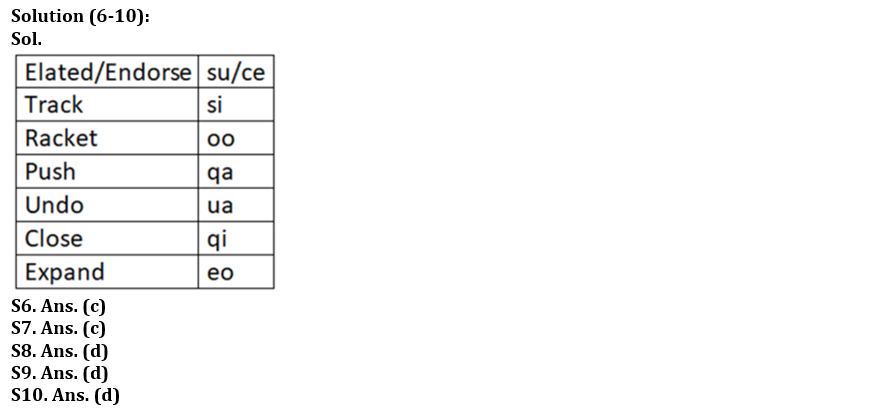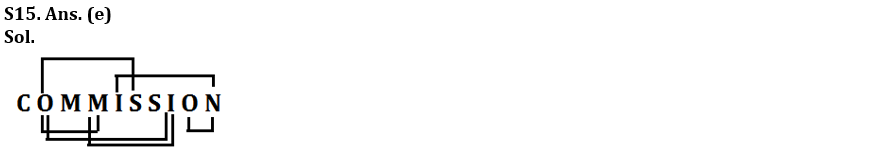Latest Banking jobs   »   Reasoning Ability Quiz For IBPS RRB...

# Reasoning Ability Quiz For IBPS RRB PO Clerk Prelims 2022- 14th August

Direction (1-5): Study the following sequence carefully and answer the questions given below:

S 5 O 4 T R 5 A 3 G 6 F D 2 E W 3 K 1 L 4 M B U 5 C X 8 Z I 9 N S 5 Q 7 Z

Q1. Which amongst the following element is the fifteenth to the right of the fifteenth element from the left end?
(a) Z
(b) I
(c) 9
(d) N
(e) None of these

Q2. How many elements are there between G and the element which is to the immediate right of X?
(a) Nineteen
(b) Eighteen
(c) Sixteen
(d) Seventeen
(e) None of these

Q3. How many numbers are there which are immediately preceded by a consonant and immediately followed by a number?
(a) None
(b) Two
(c) One
(d) More than two
(e) None of these

Q4. What is the position of ‘F’ with respect to the element which is second to the left of ‘B’?
(a) Seventh to the right
(b) Eighth to the left
(c) Ninth to the left
(d) Eighth to the right
(e) None of these

Q5. How many vowels are there which are immediately preceded by and immediately followed by a number?
(a) One
(b) Two
(c) Four
(d) Five
(e) None of these

Direction (6-10): Study the following data carefully and answer the questions accordingly.
In a certain code language,
‘Elated endorse track’ is coded as ‘su si ce’
‘Racket push undo’ is coded as ‘ua oo qa’
‘Close expand track push’ is coded as ‘eo si qa qi’
‘Racket expand elated endorse’ is coded as ‘su ce eo oo’

Q6. What is the code for ‘undo’?
(a) qa
(b) Either (a) or (d)
(c) ua
(d) oo
(e) None of these

Q7. Which of the following is coded as ‘eo’?
(a) Racket
(b) Endorse
(c) Expand
(d) Elated
(e) None of these

Q8. What is the code for ‘push racket close’?
(a) eo oo qi
(b) oo su ce
(c) qa qi ua
(d) oo qa qi
(e) None of these

Q9. Which of the following is possibly coded as ‘su eo’?
(a) Track elated
(b) Expand track
(c) Elated endorse
(d) Endorse expand
(e) Racket endorse

Q10. What is the code for ‘push’?
(a) ua
(b) si
(c) eo
(d) qa
(e) None of these

Directions (11-13): Read the following information carefully and answer the given questions:
Seven people A, C, G, K, S, M and X are having different ages. K is elder to S but younger to G. The difference between the ages of 2nd eldest and 4th youngest is 7 years. only two persons are elder to C. G is younger to M who is not the eldest. Age of K is not more than 5 years. X is elder to G but younger to C. Age of 2nd eldest person is the square of the number which is the age of K. Age difference between X and third youngest person is more than 10 years.

Q11. Who among the following is the 4th youngest?
(a) A
(b) K
(c) C
(d) S
(e) X

Q12. What is the age of M?
(a) 36
(b) 64
(c) 25
(d) 30
(e) Can’t be determined

Q13. What could be the possible age of C?
(a) 28
(b) 30
(c) 15
(d) 22
(e) None of the above

Q14. What should be placed in place of question mark(?) in equation E ≤ Z ? W ? C ? D to make Z > D always follow.
(a) =, >, ≥
(b) =, <, ≥
(c) =, >, ≤
(d) =, ≥, ≥
(e) None of these

Q15. How many pairs of letters are there in the word ‘COMMISSION’, each of which have as many letters between them (both forward and backward direction) in the word as they have between then in the English alphabet?
(a)One
(b)Three
(c) Two
(d)Four
(e) More than five

Solutions

S1. Ans. (b)
Sol. Clearly, ‘I’ is 15th to the right of 15th element from the left end.

S2. Ans. (d)
Sol. Clearly, there are seventeen elements between G and the element which is to the immediate right of X.

S3. Ans. (a)
Sol. Clearly, there is no number which is immediately preceded by a consonant and immediately followed by a number.

S4. Ans. (c)
Sol. Clearly, ‘F’ is ninth to the left of the one which is second to the left of ‘B’.

S5. Ans. (b)S11. Ans. (e)
Sol. A > M (25) > C > X (18) > G > K (5) > S

S12. Ans. (c)
Sol. A > M (25) > C > X (18) > G > K (5) > S

S13. Ans. (d)
Sol. A > M (25) > C > X (18) > G > K (5) > S

S14. Ans. (a)#### Congratulations!Download Hindu Review of October 2021: Free PDF